omG8HI9LSKFW6lhCj8prTs0Z6lUhdOT9Jhi1Sf4m

# What is Maximum Average Power Transfer Formula

We have solved the problem of maximizing the power delivered by a power-supplying resistive network to a load RL. Representing the circuit in Thevenin equivalent circuit, we proved that the maximum power would be delivered to the load if the load resistance is equal to the Thevenin resistance RL = RTh. We now extend that result to ac circuit.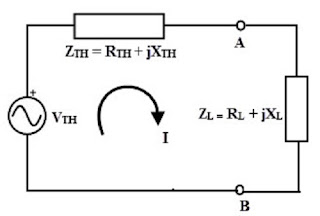Consider the circuit in Figure.(1), where an ac circuit is connected to a load ZL and is represented by its Thevenin equivalent.Figure 1. Finding the maximum average power transfer : (a) circuit with a load, (b) the Thevenin equivalent
The load is usually represented by an impedance, which may model an electric motor, an antenna, and so forth. In rectangular form, the Thevenin impedance ZTh and the load impedance ZL are(1a)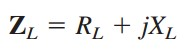(1b)
The current through the load is(2)
The average power delivered to the load is(3)
Our objective is to adjust the load parameters RL and XL so that P is maximum. To do this we set ∂P/∂RL and ∂P/∂XL equal to zero. From Equation.(3), we obtain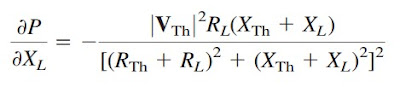(4a)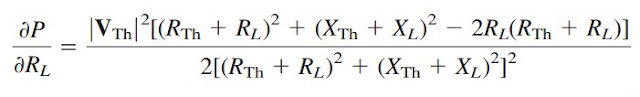(4b)
Setting ∂P/∂XL to zero gives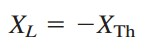(5)
and setting ∂P/∂RL to zero results(6)
Combining Equations.(5) and (6) leads to the conclusion that for maximum average power transfer, ZL must be selected so that XL = -XTh and RL = RTh, i.e,.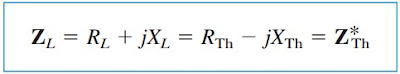(7)
For maximum average power transfer, the load impedance ZL must be equal to the complex conjugate of the Thevenin impedance ZTh.
This result is known as the maximum average power transfer theorem for the sinusoidal steady state. Setting RL = RTh and XL = -XTh in Equation.(3) gives us the maximum average power as(8)
In a situation in which the load is purely real, the condition for maximum power transfer is obtained from Equation.(6) by setting XL = 0; that is,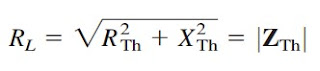(9)
This means that for maximum average power transfer to a purely resistive load, the load impedance (or resistance) is equal to the magnitude of the Thevenin impedance.

## Maximum Average Power Transfer Examples

For better understanding let us review examples below :
1. Determine the load impedance ZL that maximizes the average power drawn from the circuit of Figure.(2). What is the maximum average power?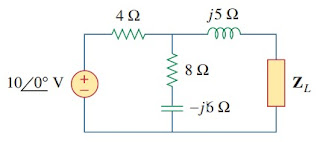Figure 2
Solution :
First we obtain the Thevenin equivalent at the load terminals. To get ZTh, consider the circuit shown in Figure.(3a). We find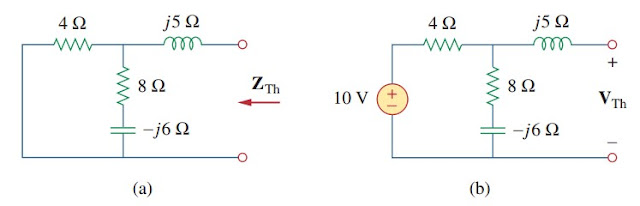Figure 3. Finding the Thevenin equivalent of the circuit in Figure.(2)
To find VTh, consider the circuit in Figure.(3b). By voltage division,
The load impedance draws the maximum power from the circuit when
According to Equation.(8), the maximum average power is

2. In the circuit in Figure.(4), find the value of RL that will absorb the maximum average power. Calculate that power.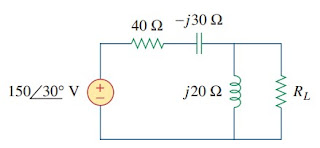Figure 4
Solution :
We first find the Thevenin equivalent at the terminals of RL.
By voltage division,
The value of RL that will absorb the maximum average power is
The current through the load is
The maximum average power absorbed by RL is

Untuk Bahasa Indonesia baca Pengertian dan Rumus Daya Rata Rata Maksimum.
Related Posts
SHARE